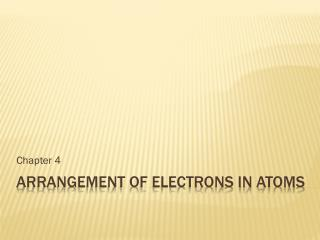Download PresentationArrangement of Electrons in Atoms

# Arrangement of Electrons in Atoms - PowerPoint PPT Presentation

Chapter 4. Arrangement of Electrons in Atoms. Light has characteristics of both particles and waves Electromagnetic radiation – a form of energy that exhibits wave-like behavior as it moves through space Electromagnetic spectrum. The Development of a New Atomic Model.I am the owner, or an agent authorized to act on behalf of the owner, of the copyrighted work described.
Download Presentation## Arrangement of Electrons in Atoms

An Image/Link below is provided (as is) to download presentation

Download Policy: Content on the Website is provided to you AS IS for your information and personal use and may not be sold / licensed / shared on other websites without getting consent from its author.While downloading, if for some reason you are not able to download a presentation, the publisher may have deleted the file from their server.

- - - - - - - - - - - - - - - - - - - - - - - - - - E N D - - - - - - - - - - - - - - - - - - - - - - - - - -
Presentation Transcript
1. Chapter 4 Arrangement of Electrons in Atoms

2. Light has characteristics of both particles and waves Electromagnetic radiation – a form of energy that exhibits wave-like behavior as it moves through space Electromagnetic spectrum The Development of a New Atomic Model

3. = wavelength (units of meters) = frequency (units of Hertz, Hz, ) = speed of light (3.00 x 108 m/s) = Planck’s constant (6.626 x 10-34 Js) Electromagnetic Spectrum

4. Ground state – the lowest energy state of an atom Excited state – when an atom has a higher potential energy than it does at its ground state Emission and Absorption • Balmer series – represents visible light region • Lyman series – represents the UV region • Paschen series – represents the infrared series

5. When a narrow beam of light is passes through a prism, it separates into specific colors in the visible spectrum. Each element has a signature spectrum. Emission Line Spectra

6. In addition to indicators in the visible spectrum, energy can be detected in the UV region as well as the infrared region. This has been scientifically observed. Emission Spectra What wavelengths represent indicators in the UV range? What about the IR range?

7. Scientists had predicted that emission spectra would be on a continuous spectrum. Is this what was scientifically observed? Emission Spectra

8. Bohr’s Model helped to explain the quantum energy levels of the atom When a photon is absorbed, the electron gains enough energy to move to an outer energy level. When an electron loses energy (in the form of a photon), energy is released. Bohr’s Model and Transition States

9. Electron Energy Transitions

10. Recap – what is the relationship between energy and frequency of a wave? What is the visible region of light? Which colors in the visible spectrum carry more energy? http://www.youtube.com/watch?v=0qKrOF-gJZ4 Photoelectric Effect

11. P.97, #1-6 (2 days to complete) Homework

12. Electrons have wave-like properties • Investigations from the photoelectric effect and hydrogen’s emission line spectra determined that light acts as both a wave and a particle. Quantum Model of the Atom

13. Electrons have interference patterns Electrons Constructive interference Destructive interference

14. http://www.youtube.com/watch?v=DfPeprQ7oGc Double Slit Experiment

15. Heisenberg’s Uncertainty Principle – you cannot know the instantaneous position and velocity of an electron (or any other particle) When you have extremely precise information about x, your uncertainty for p goes through the roof. Heisenberg x – represents position p – represents momentum (velocity multiplied by mass) - represents a constant Δ – in this case, delta represents the uncertainty.

16. Orbitals indicate probable electron locations • Schrodinger’s equation! This formed the foundation of modern quantum theory • Quantum theory – • Quantum numbers – Orbitals

17. Symbolized by n • Indicates the main energy level occupied by an electron. • True or false – these can only be integers • The total number of orbitals in a given shell is equal to n2! Principle Quantum Number

18. Different orbital shapes can exist for each principle quantum number. • Represented by l • What orbital shapes could you find at n=3? • What about when n=3 and l=2? Angular Momentum Quantum Number

19. Represented by “m” • Ranges from –l to l • Indicates the orientation of an orbital around the nucleus Magnetic Quantum Number

20. Represented by “s” Has only two possible states A single orbital can hold a maximum of two different electrons Spin Quantum Number

21. Smartboard Demonstration

22. How many electrons could be represented by n=2? How many electrons could be represented with a principle quantum number of 3 and an angular momentum quantum number of 0? How many electrons could be represented with a principle quantum number of 1 and a spin number of ½? How many electrons could be represented with a principle quantum number of 3 and a spin number of ½? How many electrons could be represented by l=3? Let’s Practice! Class Work